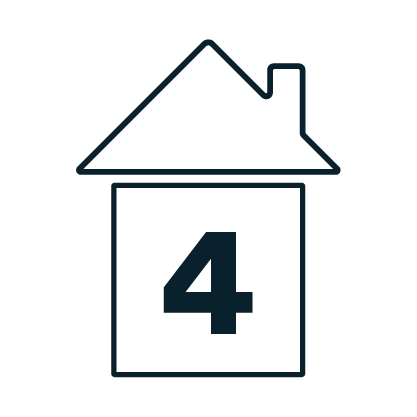##Grade 4 Module 5 Lesson 23

How can we add and multiply unit fractions to build fractions greater than 1 using visual models? In this lesson, Ms. Gradney explores adding and multiplying unit fractions using the associative property and the mathematical model of the number line. For this lesson, you’ll need paper and pencil. If you have access to a workbook, or a printer, we also suggesting using the Problem Set available using the link below the video.